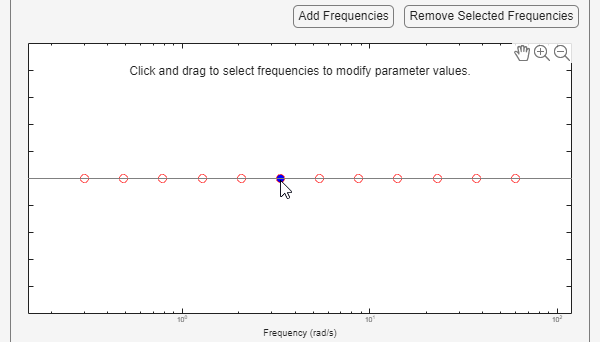## Modify Estimation Input Signals

When the frequency response estimation produces unexpected results, you can try modifying the input signal properties in the ways described in Troubleshooting Frequency Response Estimation.

### Modify Sinestream Signal Using Model Linearizer

#### Add Frequency Points to Sinestream Input Signal

This example shows how to add frequency points to an existing sinestream input signal using the Model Linearizer.

1. Create a sinestream input signal, `in_sine1`, as shown in Create Sinestream Signals Using Model Linearizer.

2. Double-click `in_sine1` in the Linear Analysis Workspace area of the Model Linearizer.

The Edit sinestream dialog box opens.

3. In the frequency content viewer, clickin the Frequency content toolbar.

The Add frequencies dialog box opens.4. Enter the frequency range of the points to be added.

5. Click to add the specified frequency points to `in_sine1`.

#### Delete Frequency Point from Sinestream Input Signal

This example shows how to delete frequency points from an existing sinestream input signal using the Model Linearizer.

1. Create a sinestream input signal, `in_sine1`, as shown in Create Sinestream Signals Using Model Linearizer.

2. Double-click `in_sine1` in the Linear Analysis Workspace area of the Model Linearizer.

The Edit sinestream dialog box opens.

3. In the frequency content viewer, select the frequency point to delete.The selected point appears blue.

Tip

To select multiple frequency points, click and drag across the frequency points of interest.

4. Clickin the Frequency content toolbar to delete the selected frequency points from the frequency content viewer.

5. Click to save the modified input signal.

#### Modify Parameters for a Frequency Point in Sinestream Input Signal

This example shows how to modify signal parameters of an existing sinestream input signal using the Model Linearizer.

1. Create a sinestream input signal, `in_sine1`, as shown in Create Sinestream Signals Using Model Linearizer.

2. Double-click `in_sine1` in the Linear Analysis Workspace area of the Model Linearizer.

The Edit sinestream dialog box opens.

3. In the frequency content viewer, select the frequency point(s) to delete.The selected point(s) appears blue.

Tip

To select multiple frequency points, click and drag across the frequency points of interest.

4. Enter the new values for the signal parameters.

If the parameter value is `<mixedvalue>`, the parameter has different values for some of the frequency points selected.

5. Click to save the modified input signal.

### Modify Sinestream Signal Using MATLAB Code

For example, suppose that you used a sinestream input signal, and the output at a specific frequency did not reach steady state. In this case, you can modify the characteristics of the sinestream input at the corresponding frequency.

```input.NumPeriods(index) = NewNumPeriods; input.SettlingPeriods(index) = NewSettlingPeriods;```

where `index` is the frequency value index of the sine wave you want to modify. `NewNumPeriods` and `NewSettlingPeriods` are the new values of `NumPeriods` and `SettlingPeriods`, respectively.

To modify several signal properties at a time, you can use the `set` command. For example:

```input = set(input,'NumPeriods',NewNumPeriods,... 'SettlingPeriods',NewSettlingPeriods)```

After modifying the input signal, repeat the estimation.